# Datasets - Reduced data, IRFs, models#

Learn how to work with datasets

## Introduction#

`datasets` are a crucial part of the gammapy API. `Dataset` objects constitute `DL4` data - binned counts, IRFs, models and the associated likelihoods. `Datasets` from the end product of the data reduction stage, see makers notebook, and are passed on to the `Fit` or estimator classes for modelling and fitting purposes.

To find the different types of `Dataset` objects that are supported see Datasets home

## Setup#

```import numpy as np
import astropy.units as u
from astropy.coordinates import SkyCoord
from regions import CircleSkyRegion
import matplotlib.pyplot as plt
from gammapy.data import GTI
from gammapy.datasets import (
Datasets,
FluxPointsDataset,
MapDataset,
SpectrumDatasetOnOff,
)
from gammapy.estimators import FluxPoints
from gammapy.maps import MapAxis, WcsGeom
from gammapy.modeling.models import FoVBackgroundModel, PowerLawSpectralModel, SkyModel
```

## Check setup#

```from gammapy.utils.check import check_tutorials_setup
from gammapy.utils.scripts import make_path

# %matplotlib inline

check_tutorials_setup()
```
```System:

python_executable      : /home/runner/micromamba-root/envs/gammapy-dev/bin/python
python_version         : 3.8.13
machine                : x86_64
system                 : Linux

Gammapy package:

version                : 0.20.2.dev535+g4ab0530ce
path                   : /home/runner/work/gammapy-docs/gammapy-docs/gammapy/gammapy

Other packages:

numpy                  : 1.22.4
scipy                  : 1.9.1
astropy                : 5.1
regions                : 0.6
click                  : 8.1.3
yaml                   : 6.0
IPython                : 8.5.0
jupyterlab             : 3.4.7
matplotlib             : 3.6.0
pandas                 : 1.5.0
healpy                 : 1.16.1
iminuit                : 2.16.0
sherpa                 : 4.14.1
naima                  : 0.10.0
emcee                  : 3.1.2
corner                 : 2.2.1

Gammapy environment variables:

GAMMAPY_DATA           : /home/runner/work/gammapy-docs/gammapy-docs/gammapy-datasets
```

## MapDataset#

The counts, exposure, background, masks, and IRF maps are bundled together in a data structure named `MapDataset`. While the `counts`, and `background` maps are binned in reconstructed energy and must have the same geometry, the IRF maps can have a different spatial (coarsely binned and larger) geometry and spectral range (binned in true energies). It is usually recommended that the true energy bin should be larger and more finely sampled and the reco energy bin.

### Creating an empty dataset#

An empty `MapDataset` can be directly instantiated from any `WcsGeom` object:

```energy_axis = MapAxis.from_energy_bounds(1, 10, nbin=11, name="energy", unit="TeV")

geom = WcsGeom.create(
skydir=(83.63, 22.01),
axes=[energy_axis],
width=5 * u.deg,
binsz=0.05 * u.deg,
frame="icrs",
)

dataset_empty = MapDataset.create(geom=geom, name="my-dataset")
```

It is good practice to define a name for the dataset, such that you can identify it later by name. However if you define a name it must be unique. Now we can already print the dataset:

```print(dataset_empty)
```
```MapDataset
----------

Name                            : my-dataset

Total counts                    : 0
Total background counts         : 0.00
Total excess counts             : 0.00

Predicted counts                : 0.00
Predicted background counts     : 0.00
Predicted excess counts         : nan

Exposure min                    : 0.00e+00 m2 s
Exposure max                    : 0.00e+00 m2 s

Number of total bins            : 110000
Number of fit bins              : 0

Fit statistic type              : cash
Fit statistic value (-2 log(L)) : nan

Number of models                : 0
Number of parameters            : 0
Number of free parameters       : 0
```

The printout shows the key summary information of the dataset, such as total counts, fit statistics, model information etc.

`from_geom` has additional keywords, that can be used to define the binning of the IRF related maps:

```# choose a different true energy binning for the exposure, psf and edisp
energy_axis_true = MapAxis.from_energy_bounds(
0.1, 100, nbin=11, name="energy_true", unit="TeV", per_decade=True
)

# choose a different rad axis binning for the psf
rad_axis = MapAxis.from_bounds(0, 5, nbin=50, unit="deg", name="rad")

gti = GTI.create(0 * u.s, 1000 * u.s)

dataset_empty = MapDataset.create(
geom=geom,
energy_axis_true=energy_axis_true,
binsz_irf=0.1,
gti=gti,
name="dataset-empty",
)
```

To see the geometry of each map, we can use:

```dataset_empty.geoms
```

Another way to create a `MapDataset` is to just read an existing one from a FITS file:

```dataset_cta = MapDataset.read(
"\$GAMMAPY_DATA/cta-1dc-gc/cta-1dc-gc.fits.gz", name="dataset-cta"
)

print(dataset_cta)
```
```MapDataset
----------

Name                            : dataset-cta

Total counts                    : 104317
Total background counts         : 91507.70
Total excess counts             : 12809.30

Predicted counts                : 91507.69
Predicted background counts     : 91507.70
Predicted excess counts         : nan

Exposure min                    : 6.28e+07 m2 s
Exposure max                    : 1.90e+10 m2 s

Number of total bins            : 768000
Number of fit bins              : 691680

Fit statistic type              : cash
Fit statistic value (-2 log(L)) : nan

Number of models                : 0
Number of parameters            : 0
Number of free parameters       : 0
```

## Accessing contents of a dataset#

To further explore the contents of a `Dataset`, you can use e.g. `info_dict()`

```# For a quick info, use
dataset_cta.info_dict()

# For a quick view, use
dataset_cta.peek()
```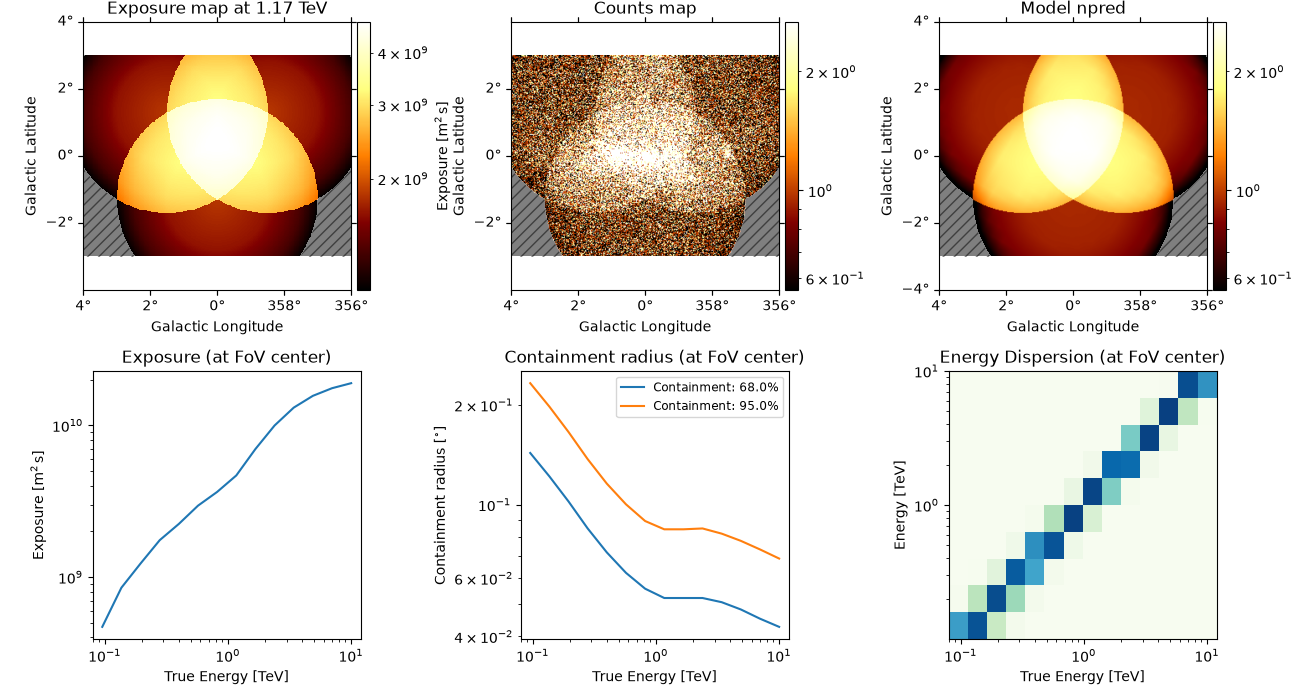And access individual maps like:

```counts_image = dataset_cta.counts.sum_over_axes()
counts_image.smooth("0.1 deg").plot()
```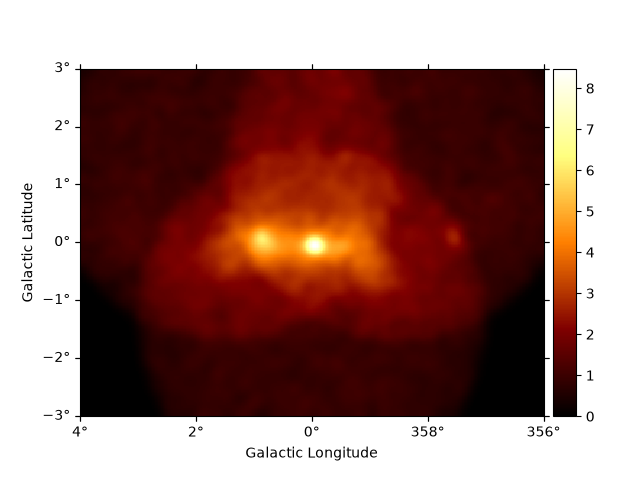Of course you can also access IRF related maps, e.g. the psf as `PSFMap`:

```dataset_cta.psf
```

And use any method on the `PSFMap` object:

```radius = dataset_cta.psf.containment_radius(energy_true=1 * u.TeV, fraction=0.95)

ax = plt.subplot()
edisp_kernel = dataset_cta.edisp.get_edisp_kernel()
edisp_kernel.plot_matrix(ax=ax)
```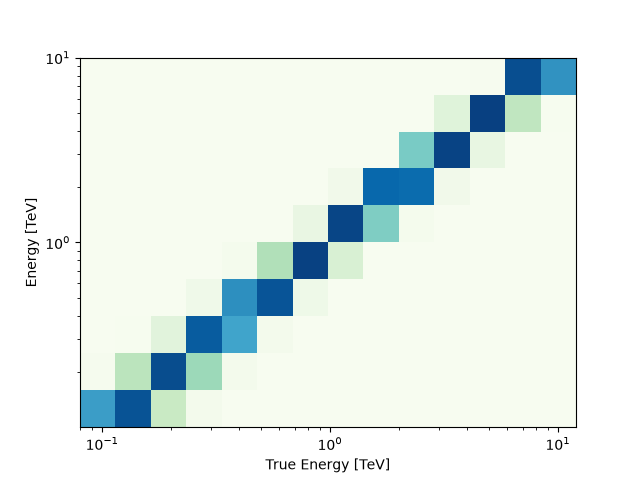```[0.08675] deg
```

The `MapDataset` typically also contains the information on the residual hadronic background, stored in `background` as a map:

```dataset_cta.background
```

As a next step we define a minimal model on the dataset using the `models` setter:

```model = SkyModel.create("pl", "point", name="gc")
model.spatial_model.position = SkyCoord("0d", "0d", frame="galactic")
model_bkg = FoVBackgroundModel(dataset_name="dataset-cta")

dataset_cta.models = [model, model_bkg]
```

Assigning models to datasets is covered in more detail in the Modeling notebook. Printing the dataset will now show the mode components:

```print(dataset_cta)
```
```MapDataset
----------

Name                            : dataset-cta

Total counts                    : 104317
Total background counts         : 91507.70
Total excess counts             : 12809.30

Predicted counts                : 91719.65
Predicted background counts     : 91507.69
Predicted excess counts         : 211.96

Exposure min                    : 6.28e+07 m2 s
Exposure max                    : 1.90e+10 m2 s

Number of total bins            : 768000
Number of fit bins              : 691680

Fit statistic type              : cash
Fit statistic value (-2 log(L)) : 424648.57

Number of models                : 2
Number of parameters            : 8
Number of free parameters       : 5

Component 0: SkyModel

Name                      : gc
Datasets names            : None
Spectral model type       : PowerLawSpectralModel
Spatial  model type       : PointSpatialModel
Temporal model type       :
Parameters:
index                         :      2.000   +/-    0.00
amplitude                     :   1.00e-12   +/- 0.0e+00 1 / (cm2 s TeV)
reference             (frozen):      1.000       TeV
lon_0                         :      4.650   +/-    0.00 rad
lat_0                         :     -0.505   +/-    0.00 rad

Component 1: FoVBackgroundModel

Name                      : dataset-cta-bkg
Datasets names            : ['dataset-cta']
Spectral model type       : PowerLawNormSpectralModel
Parameters:
norm                          :      1.000   +/-    0.00
tilt                  (frozen):      0.000
reference             (frozen):      1.000       TeV
```

Now we can use the `npred` method to get a map of the total predicted counts of the model:

```npred = dataset_cta.npred()
npred.sum_over_axes().plot()
```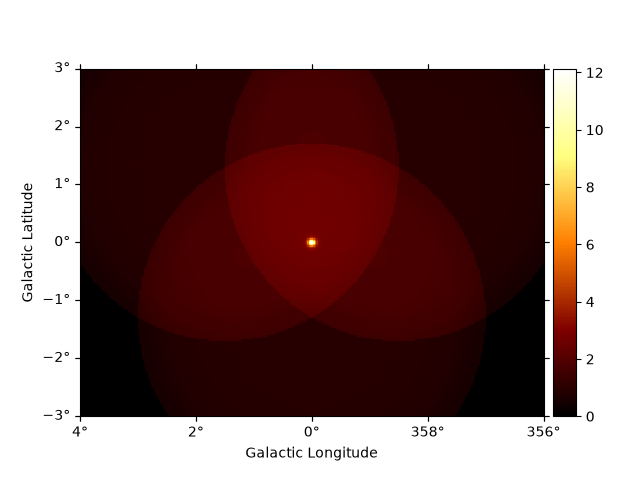To get the predicted counts from an individual model component we can use:

```npred_source = dataset_cta.npred_signal(model_name="gc")
npred_source.sum_over_axes().plot()
```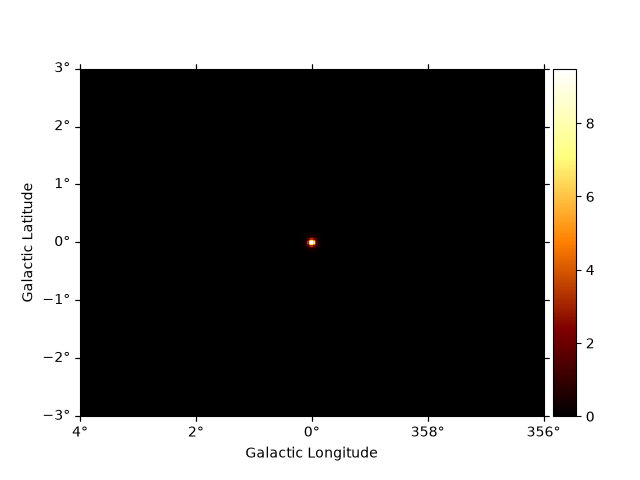`background` contains the background map computed from the IRF. Internally it will be combined with a `FoVBackgroundModel`, to allow for adjusting the backgroun model during a fit. To get the model corrected background, one can use `npred_background`.

```npred_background = dataset_cta.npred_background()
npred_background.sum_over_axes().plot()
```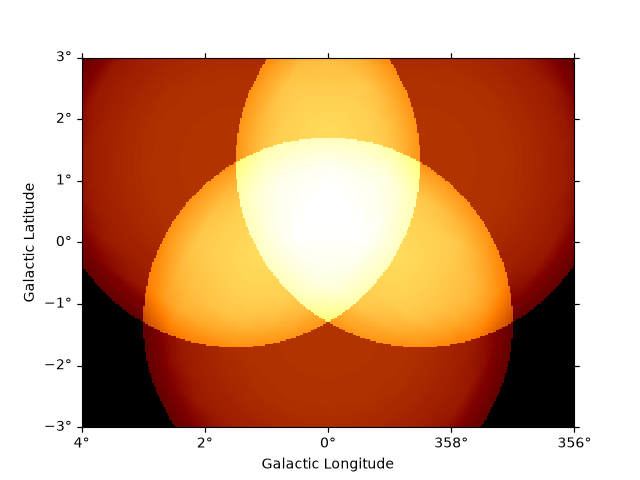There are two masks that can be set on a `MapDataset`, `mask_safe` and `mask_fit`.

• The `mask_safe` is computed during the data reduction process according to the specified selection cuts, and should not be changed by the user.

• During modelling and fitting, the user might want to additionally ignore some parts of a reduced dataset, e.g. to restrict the fit to a specific energy range or to ignore parts of the region of interest. This should be done by applying the `mask_fit`. To see details of applying masks, please refer to Masks for fitting.

Both the `mask_fit` and `mask_safe` must have the same `geom` as the `counts` and `background` maps.

```# eg: to see the safe data range
```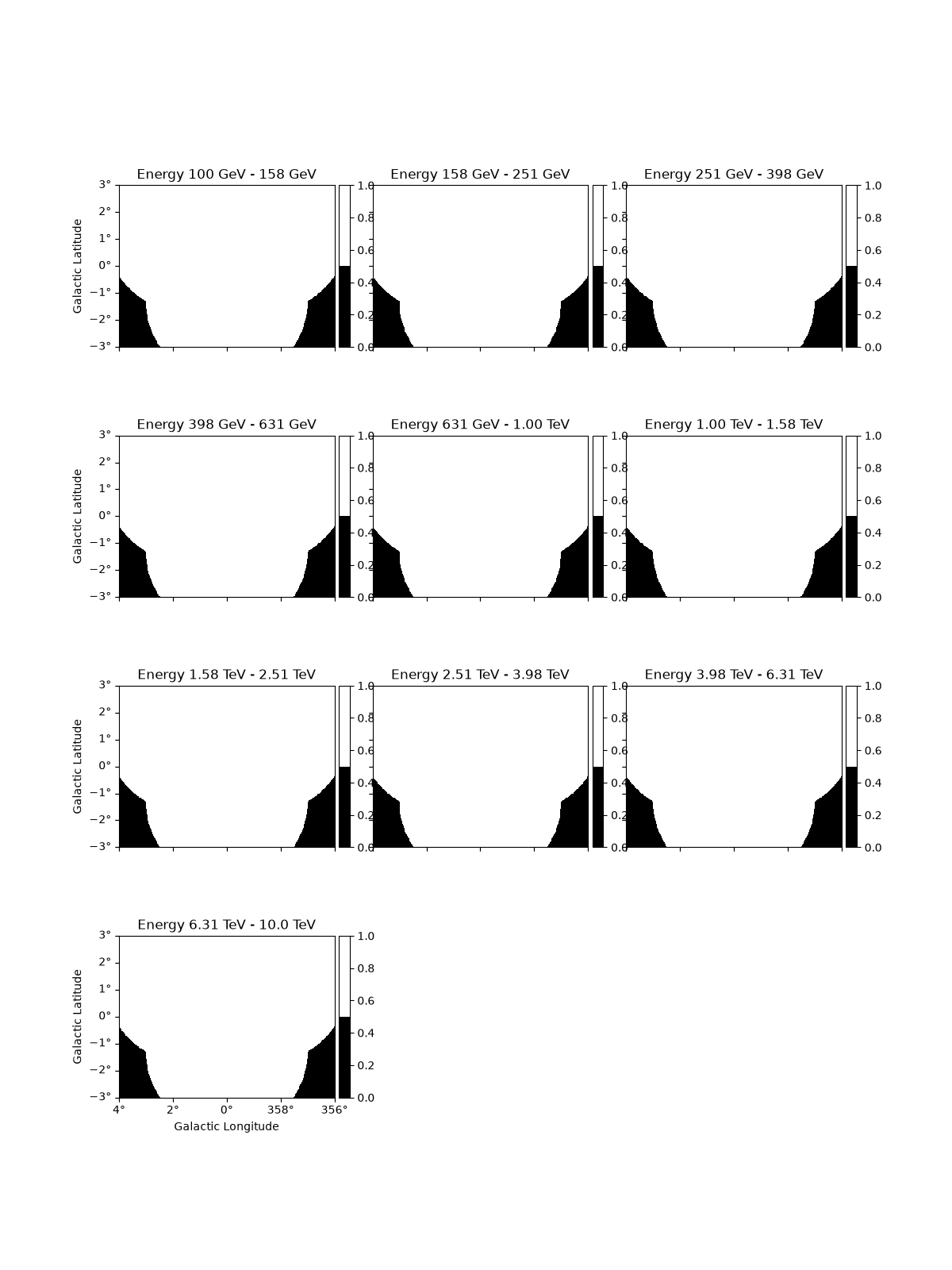In addition it is possible to define a custom `mask_fit`:

```# To apply a mask fit - in enegy and space
region = CircleSkyRegion(SkyCoord("0d", "0d", frame="galactic"), 1.5 * u.deg)

geom = dataset_cta.counts.geom

mask_energy = geom.energy_mask(0.3 * u.TeV, 8 * u.TeV)
```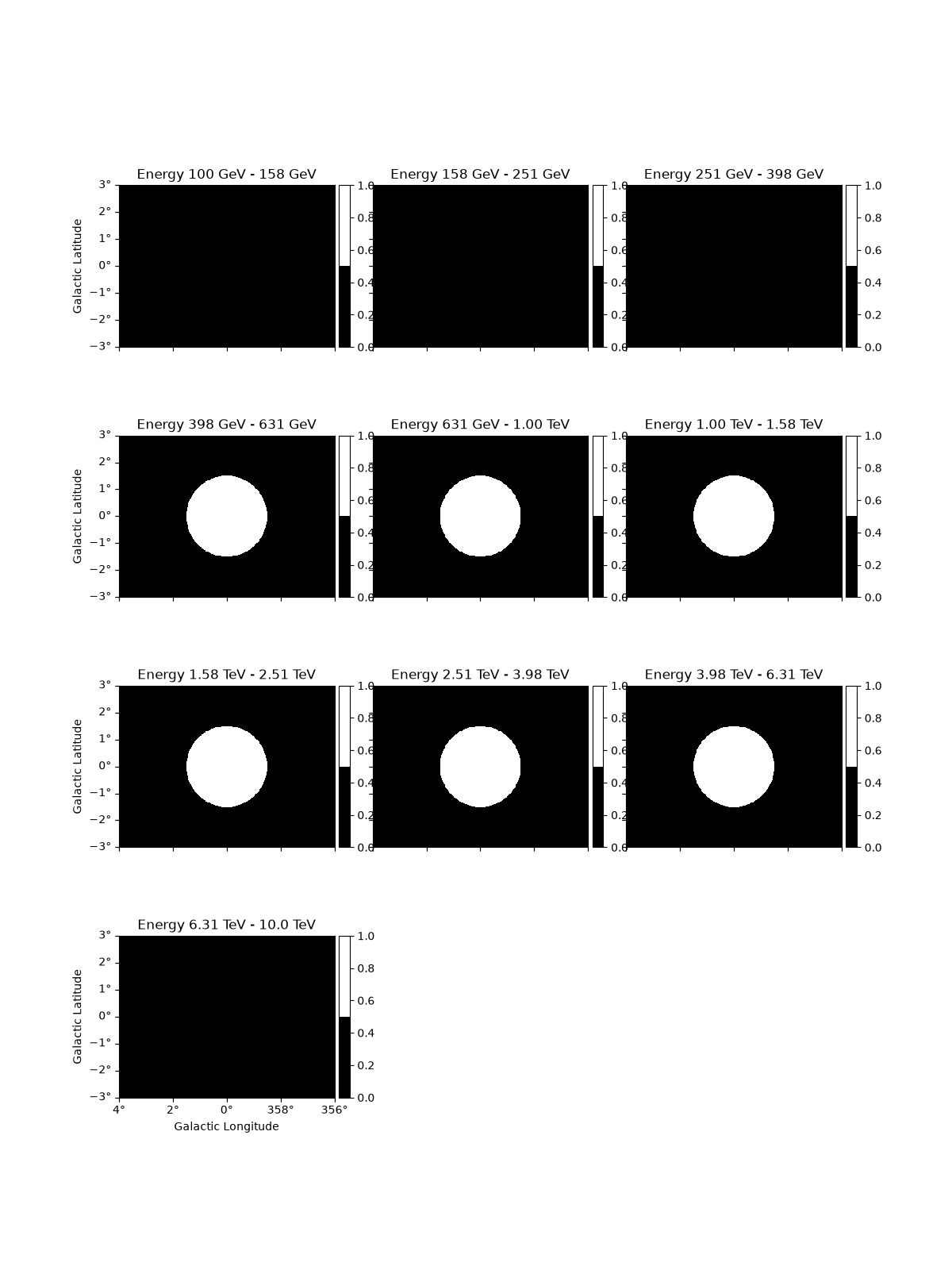To access the energy range defined by the mask you can use:

-`energy_range_safe` : energy range definedby the `mask_safe` - `energy_range_fit` : energy range defined by the `mask_fit` - `energy_range` : the final energy range used in likelihood computation

These methods return two maps, with the `min` and `max` energy values at each spatial pixel

```e_min, e_max = dataset_cta.energy_range

# To see the lower energy threshold at each point

# To see the lower energy threshold at each point
```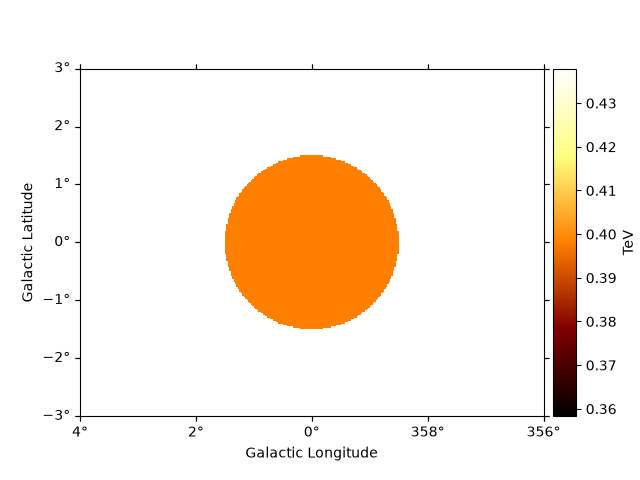Just as for `Map` objects it is possible to cutout a whole `MapDataset`, which will perform the cutout for all maps in parallel.Optionally one can provide a new name to the resulting dataset:

```cutout = dataset_cta.cutout(
position=SkyCoord("0d", "0d", frame="galactic"),
width=2 * u.deg,
name="cta-cutout",
)

cutout.counts.sum_over_axes().plot()
```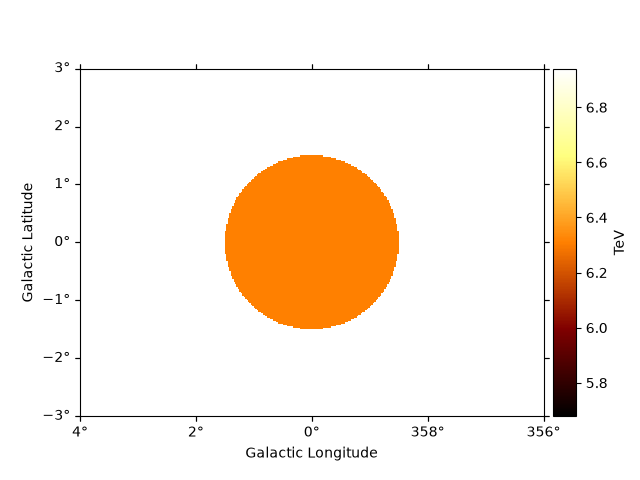It is also possible to slice a `MapDataset` in energy:

```sliced = dataset_cta.slice_by_energy(
energy_min=1 * u.TeV, energy_max=5 * u.TeV, name="slice-energy"
)
sliced.counts.plot_grid()
```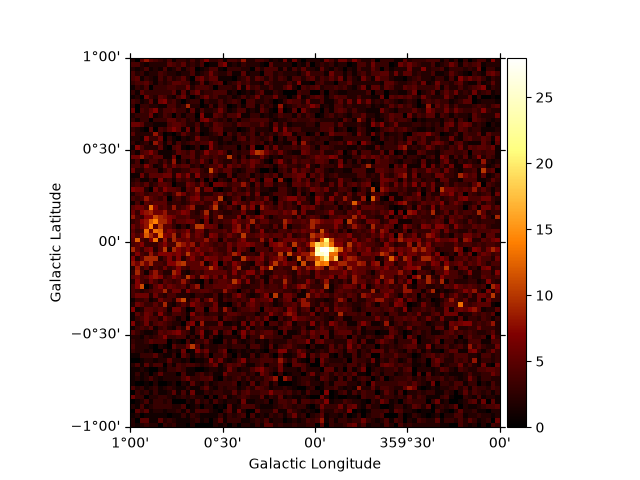The same operation will be applied to all other maps contained in the datasets such as `mask_fit`:

```sliced.mask_fit.plot_grid()
```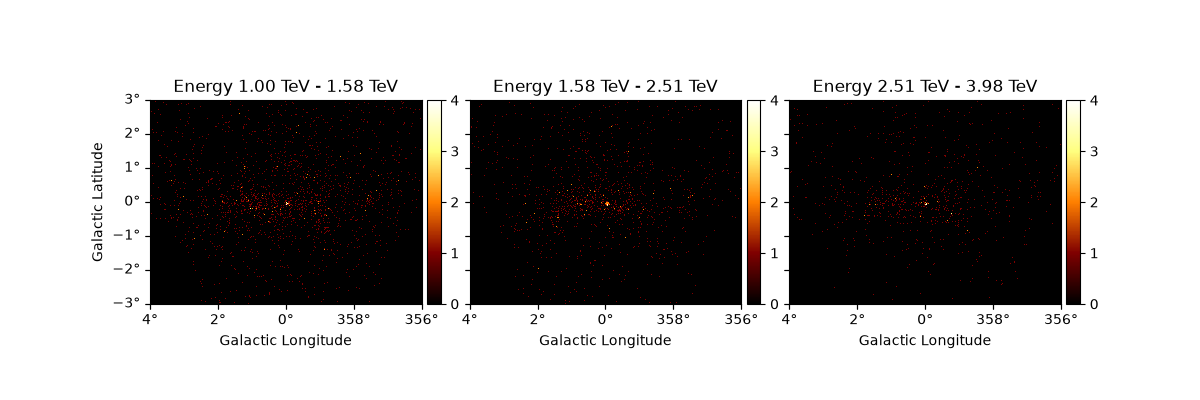### Resampling datasets#

It can often be useful to coarsely rebin an initially computed datasets by a specified factor. This can be done in either spatial or energy axes:

```downsampled = dataset_cta.downsample(factor=8)
downsampled.counts.sum_over_axes().plot()
```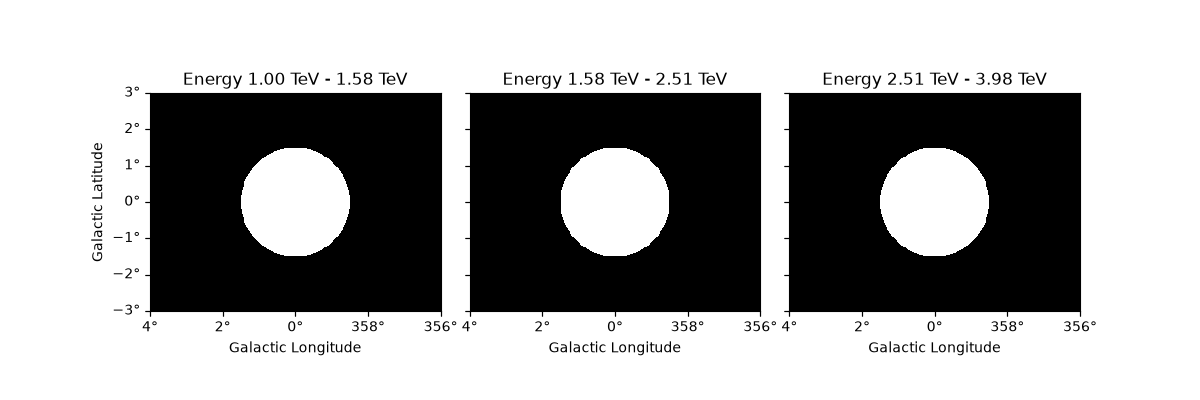And the same downsampling process is possible along the energy axis:

```downsampled_energy = dataset_cta.downsample(
factor=5, axis_name="energy", name="downsampled-energy"
)
downsampled_energy.counts.plot_grid()
```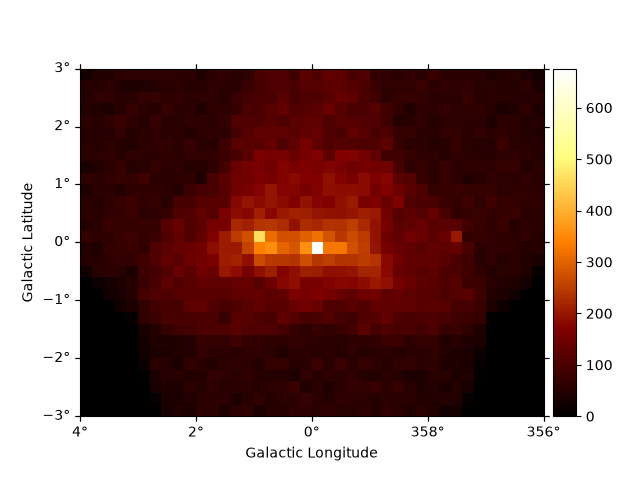In the printout one can see that the actual number of counts is preserved during the downsampling:

```print(downsampled_energy, dataset_cta)
```
```MapDataset
----------

Name                            : downsampled-energy

Total counts                    : 104317
Total background counts         : 91507.69
Total excess counts             : 12809.31

Predicted counts                : 91507.69
Predicted background counts     : 91507.69
Predicted excess counts         : nan

Exposure min                    : 6.28e+07 m2 s
Exposure max                    : 1.90e+10 m2 s

Number of total bins            : 153600
Number of fit bins              : 22608

Fit statistic type              : cash
Fit statistic value (-2 log(L)) : nan

Number of models                : 0
Number of parameters            : 0
Number of free parameters       : 0

MapDataset
----------

Name                            : dataset-cta

Total counts                    : 104317
Total background counts         : 91507.70
Total excess counts             : 12809.30

Predicted counts                : 91719.65
Predicted background counts     : 91507.69
Predicted excess counts         : 211.96

Exposure min                    : 6.28e+07 m2 s
Exposure max                    : 1.90e+10 m2 s

Number of total bins            : 768000
Number of fit bins              : 67824

Fit statistic type              : cash
Fit statistic value (-2 log(L)) : 44317.66

Number of models                : 2
Number of parameters            : 8
Number of free parameters       : 5

Component 0: SkyModel

Name                      : gc
Datasets names            : None
Spectral model type       : PowerLawSpectralModel
Spatial  model type       : PointSpatialModel
Temporal model type       :
Parameters:
index                         :      2.000   +/-    0.00
amplitude                     :   1.00e-12   +/- 0.0e+00 1 / (cm2 s TeV)
reference             (frozen):      1.000       TeV
lon_0                         :      4.650   +/-    0.00 rad
lat_0                         :     -0.505   +/-    0.00 rad

Component 1: FoVBackgroundModel

Name                      : dataset-cta-bkg
Datasets names            : ['dataset-cta']
Spectral model type       : PowerLawNormSpectralModel
Parameters:
norm                          :      1.000   +/-    0.00
tilt                  (frozen):      0.000
reference             (frozen):      1.000       TeV
```

We can also resample the finer binned datasets to an arbitrary coarser energy binning using:

```energy_axis_new = MapAxis.from_energy_edges([0.1, 0.3, 1, 3, 10] * u.TeV)
resampled = dataset_cta.resample_energy_axis(energy_axis=energy_axis_new)
resampled.counts.plot_grid(ncols=2)
```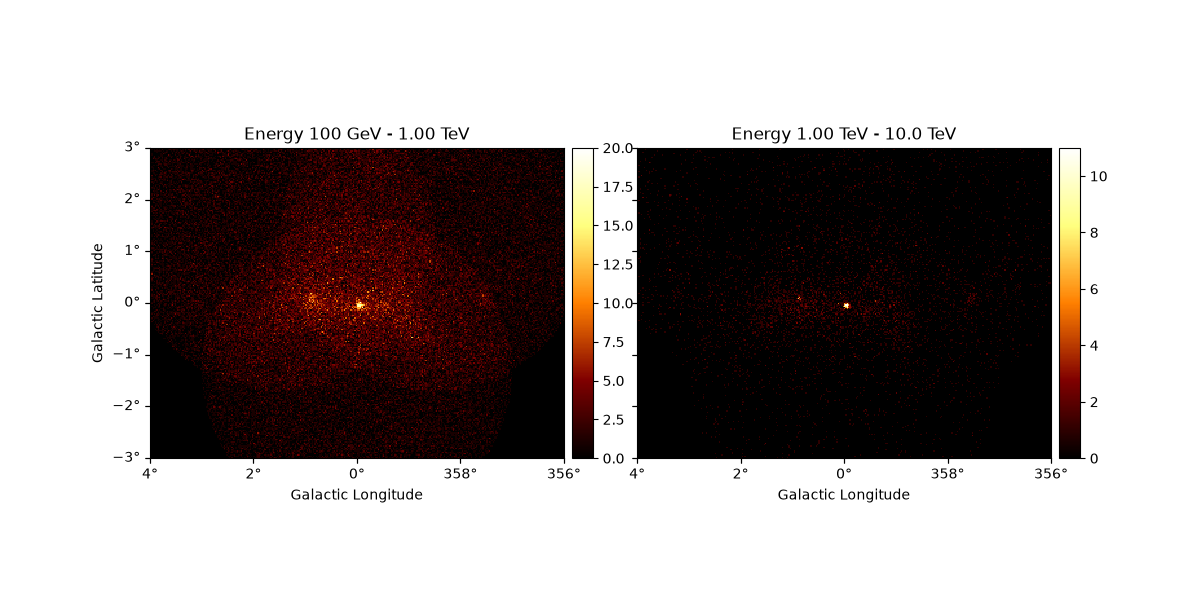To squash the whole dataset into a single energy bin there is the `to_image()` convenience method:

```dataset_image = dataset_cta.to_image()
dataset_image.counts.plot()
```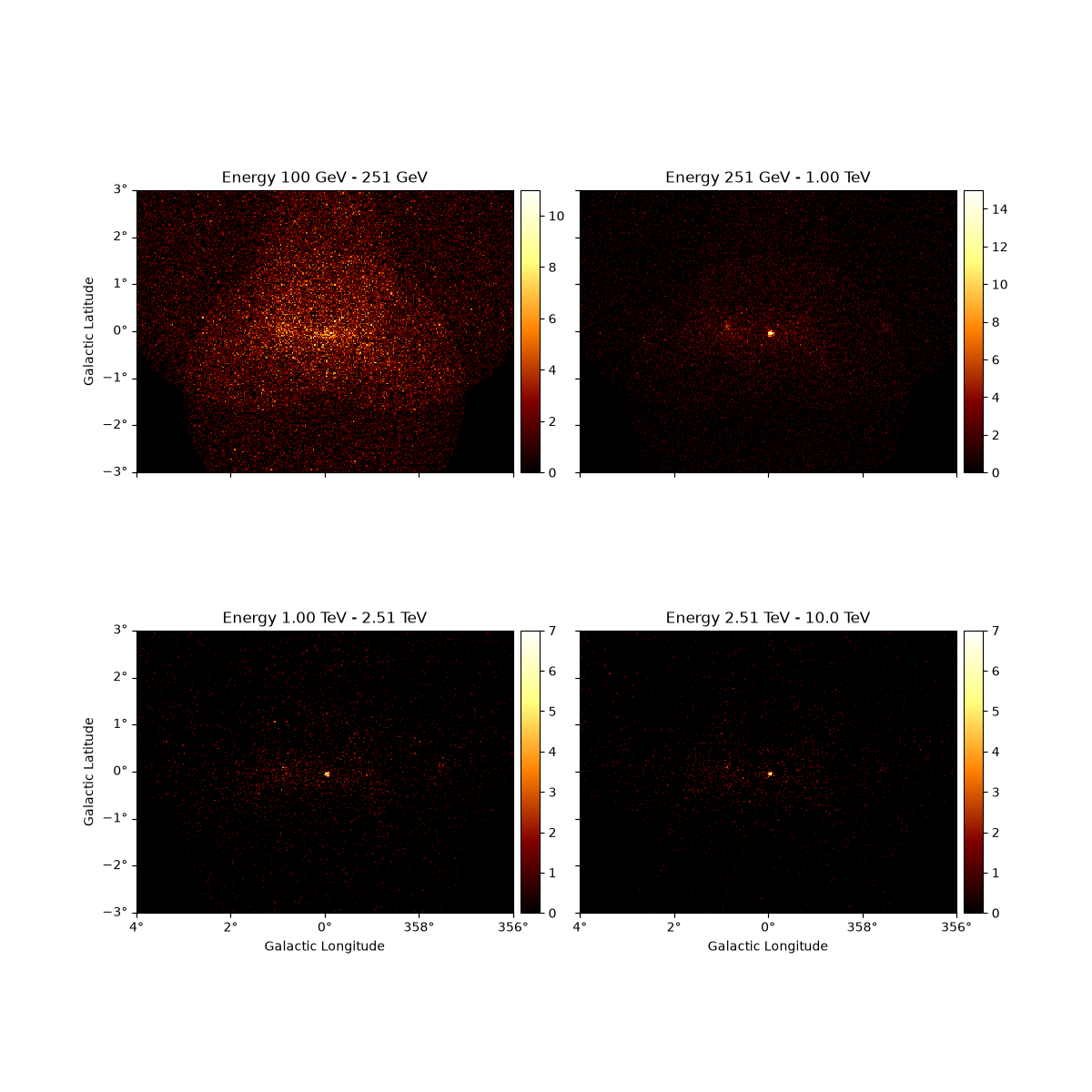## SpectrumDataset#

`SpectrumDataset` inherits from a `MapDataset`, and is specially adapted for 1D spectral analysis, and uses a `RegionGeom` instead of a `WcsGeom`. A `MapDatset` can be converted to a `SpectrumDataset`, by summing the `counts` and `background` inside the `on_region`, which can then be used for classical spectral analysis. Containment correction is feasible only for circular regions.

```region = CircleSkyRegion(SkyCoord(0, 0, unit="deg", frame="galactic"), 0.5 * u.deg)
spectrum_dataset = dataset_cta.to_spectrum_dataset(
region, containment_correction=True, name="spectrum-dataset"
)

# For a quick look
spectrum_dataset.peek()
```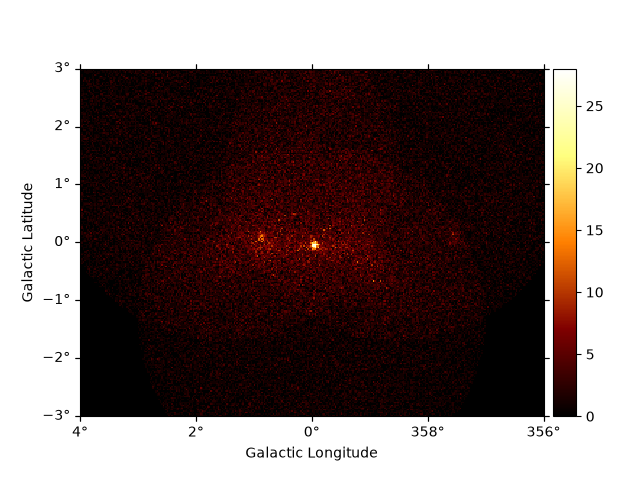A `MapDataset` can also be integrated over the `on_region` to create a `MapDataset` with a `RegionGeom`. Complex regions can be handled and since the full IRFs are used, containment correction is not required.

```reg_dataset = dataset_cta.to_region_map_dataset(region, name="region-map-dataset")
print(reg_dataset)
```
```MapDataset
----------

Name                            : region-map-dataset

Total counts                    : 5644
Total background counts         : 3323.66
Total excess counts             : 2320.34

Predicted counts                : 3323.66
Predicted background counts     : 3323.66
Predicted excess counts         : nan

Exposure min                    : 4.66e+08 m2 s
Exposure max                    : 1.89e+10 m2 s

Number of total bins            : 10
Number of fit bins              : 6

Fit statistic type              : cash
Fit statistic value (-2 log(L)) : nan

Number of models                : 0
Number of parameters            : 0
Number of free parameters       : 0
```

## FluxPointsDataset#

`FluxPointsDataset` is a `Dataset` container for precomputed flux points, which can be then used in fitting. `FluxPointsDataset` cannot be read directly, but should be read through `FluxPoints`, with an additional `SkyModel`. Similarly, `write` only saves the `FluxPointsDataset,data` attribute to disk.

```flux_points = FluxPoints.read(
"\$GAMMAPY_DATA/tests/spectrum/flux_points/diff_flux_points.fits"
)
model = SkyModel(spectral_model=PowerLawSpectralModel(index=2.3))
fp_dataset = FluxPointsDataset(data=flux_points, models=model)

fp_dataset.plot_spectrum()
```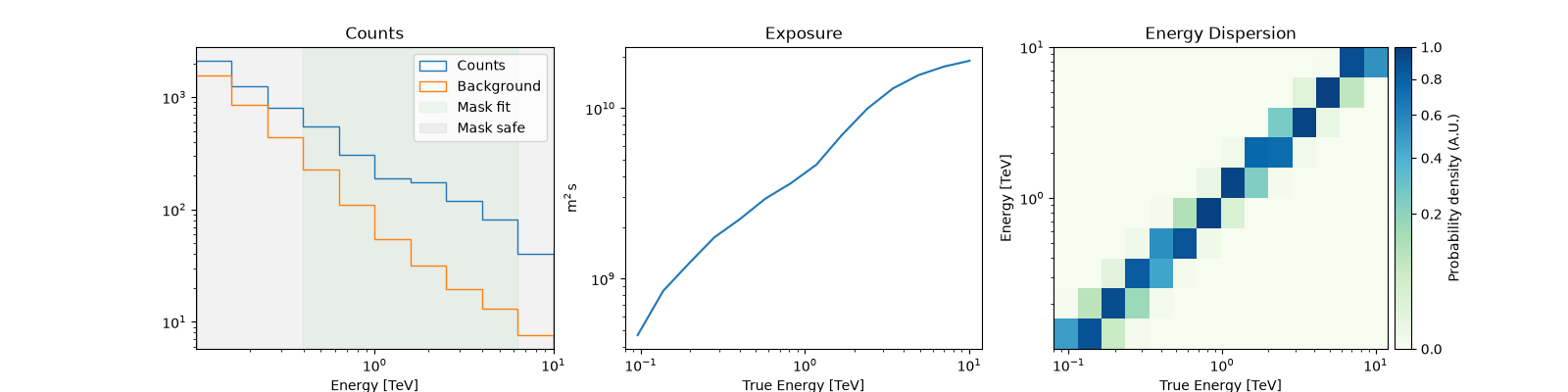The masks on `FluxPointsDataset` are `ndarray` objects and the data is a `FluxPoints` object. The `mask_safe`, by default, masks the upper limit points

```fp_dataset.mask_safe  # Note: the mask here is simply a numpy array

fp_dataset.data  # is a `FluxPoints` object

fp_dataset.data_shape()  # number of data points
```

For an example of fitting `FluxPoints`, see flux point fitting, and can be used for catalog objects, eg see catalog notebook

## Datasets#

`Datasets` are a collection of `Dataset` objects. They can be of the same type, or of different types, eg: mix of `FluxPointDataset`, `MapDataset` and `SpectrumDataset`.

For modelling and fitting of a list of `Dataset` objects, you can either - Do a joint fitting of all the datasets together - Stack the datasets together, and then fit them.

`Datasets` is a convenient tool to handle joint fitting of simultaneous datasets. As an example, please see the joint fitting tutorial

To see how stacking is performed, please see Implementation of stacking

To create a `Datasets` object, pass a list of `Dataset` on init, eg

```datasets = Datasets([dataset_empty, dataset_cta])

print(datasets)
```
```Datasets
--------

Dataset 0:

Type       : MapDataset
Name       : dataset-empty
Instrument :
Models     :

Dataset 1:

Type       : MapDataset
Name       : dataset-cta
Instrument :
Models     : ['gc', 'dataset-cta-bkg']
```

If all the datasets have the same type we can also print an info table, collectiong all the information from the individual calls to `info_dict()`:

```datasets.info_table()  # quick info of all datasets

datasets.names  # unique name of each dataset
```

We can access individual datasets in `Datasets` object by name:

```datasets["dataset-empty"]  # extracts the first dataset
```

Or by index:

```datasets
```

Other list type operations work as well such as:

```# Use python list convention to remove/add datasets, eg:
datasets.remove("dataset-empty")
datasets.names
```

Or

```datasets.append(spectrum_dataset)
datasets.names
```

Let’s create a list of spectrum datasets to illustrate some more functionality:

```datasets = Datasets()

path = make_path("\$GAMMAPY_DATA/joint-crab/spectra/hess")

for filename in path.glob("pha_*.fits"):
datasets.append(dataset)

print(datasets)
```
```Datasets
--------

Dataset 0:

Type       : SpectrumDatasetOnOff
Name       : 23526
Instrument :
Models     :

Dataset 1:

Type       : SpectrumDatasetOnOff
Name       : 23523
Instrument :
Models     :

Dataset 2:

Type       : SpectrumDatasetOnOff
Name       : 23592
Instrument :
Models     :

Dataset 3:

Type       : SpectrumDatasetOnOff
Name       : 23559
Instrument :
Models     :
```

Now we can stack all datasets using `stack_reduce()`:

```stacked = datasets.stack_reduce(name="stacked")
print(stacked)
```
```SpectrumDatasetOnOff
--------------------

Name                            : stacked

Total counts                    : 459
Total background counts         : 27.50
Total excess counts             : 431.50

Predicted counts                : 45.27
Predicted background counts     : 45.27
Predicted excess counts         : nan

Exposure min                    : 2.13e+06 m2 s
Exposure max                    : 2.63e+09 m2 s

Number of total bins            : 80
Number of fit bins              : 43

Fit statistic type              : wstat
Fit statistic value (-2 log(L)) : 1530.36

Number of models                : 0
Number of parameters            : 0
Number of free parameters       : 0

Total counts_off                : 645
Acceptance                      : 80
Acceptance off                  : 2016
```

Or slice all datasets by a given energy range:

```datasets_sliced = datasets.slice_by_energy(energy_min="1 TeV", energy_max="10 TeV")
print(datasets_sliced.energy_ranges)
```
```(<Quantity [1.e+09, 1.e+09, 1.e+09, 1.e+09] keV>, <Quantity [1.e+10, 1.e+10, 1.e+10, 1.e+10] keV>)
```

Total running time of the script: ( 0 minutes 15.554 seconds)

Gallery generated by Sphinx-Gallery